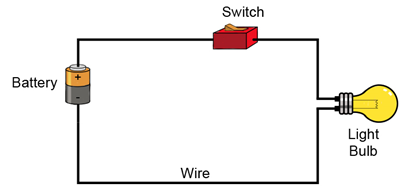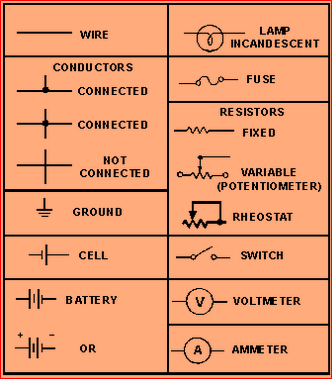# NCERT Class 10: Science Learning Concepts

### Science: Chapter 12-Electricity-Concepts

12.3 Circuit Diagram
Electric current is a stream of electrical charges. If there is a net positive charge at some place in a conductor, the charges flow immediately to the lower potential until the unbalanced charge is neutralised, and the charge flow stops. To maintain a steady flow of charge, we need a source of energy or potential difference, a load-usually an electric appliance where the energy is spent, and a conducting wire along which the charge would flow. All these elements must form a continuous path or loop so that the principle of conservation of charge is not violated. When we connect the two terminals of a cell with a conducting wire, the potential difference of the cell sets the charges in motion. A charge starts from the positive plate of a cell with a certain energy, travels through the wire to the load, where the charge expends its energy, and finally enters at the negative terminal of the cell, again picks up energy,
and the process repeats. In this way the total number of charges in the system remains the same. The current simply conveys the electrical energy along the circuit to an electric appliance that uses the energy to do work. This continuous conducting path along which the electric current flows is called an electric circuit. Fundamentally, an electric circuit is a mean of conveying electrical energy.A continuous conducting path of electric current is also called a closed electric circuit. A steady flow of current only occurs if the path is continuous. If the path is broken at any point, the current flow stops and the circuit is said to be an open. For the convenient operation of a circuit, a switch or a plug key is used to open or close the circuit. If the switch is on or the key is closed then the circuit is said to be closed. If the switch is off or the key is removed, then the circuit is open. A simple electric circuit has the following elements-a cell, connecting wires, an electric bulb (the load), a switch, an ammeter, and a voltmeter. These are the circuit elements. Each element is represented by a symbol. The symbols are used to draw a simple diagram on a paper showing how various elements are connected in a circuit-called the circuit diagram. A circuit diagram is very useful in calculations involving electricity and electronics. The following table gives a list of different electrical elements and their symbols.There is also a type of circuit called short circuit. In a short circuit, the two parts of the conducting path, one which starts from the positive terminal and the other which starts from the negative terminal, if somehow bypass the load, and come in contact with each other. The charges then cannot get rid of their energy and therefore the circuit becomes unusually hot and may cause fire.